Disclaimer: This is an example of a student written essay.

Any scientific information contained within this essay should not be treated as fact, this content is to be used for educational purposes only and may contain factual inaccuracies or be out of date.

# Investigating the Accuracy of the Air-column Length

 ✅ Paper Type: Free Essay ✅ Subject: Physics ✅ Wordcount: 2251 words ✅ Published: 9th Mar 2018

1.0 Introduction (max 500 words)

Sound is a vibration that transmits as a typically audible mechanical wave of displacement and pressure through a medium such as water or air (Wikipedia, 2015). A pressure disturbance that travels through a medium (particle to particle interaction) is called a sound wave. Particles become disturbed, causing one to exert a force to the adjacent particle; hence particles are disturbed from rest resulting in energy being transported through the medium (The Physics Classroom, 1996-2015). The displacement of a medium’s particles is parallel to the wave propagation direction, which characterises longitudinal wave (A.Russell D, 1998). Particles do not travel down the tube; they vibrate back and forth between their individual equilibrium. Due to the longitudinal waves, there are particles in the air that are compressed, whereas others are dispersed, which are called compression and rarefaction (Figure 1). The longitudinal wave does not have peaks, so the wavelength is usually measured using the distance between the compressions or the rarefactions.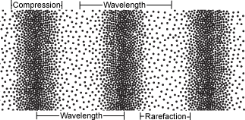Figure 1 – Compression and rarefaction (Geeks Unite, 2013)

The speed of sound is dependent on the temperature, which can be generated from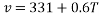, where v is the speed of sound and T is the temperature. However, in the experiment the velocity of sound waves is found by multiplying the wavelength and the known frequency of tuning forks.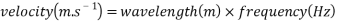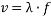Wavelength is described as the length of one cycle of the wave. When a system is driven by the natural frequency, a resonance frequency occurs. However, the amount of wavelength sections can determine several natural frequencies through a column of air in a tube (in Figure 2). When a tuning fork is held above the tube (Figure 2) while vibrating, if the tuning fork’s frequency aligns with the air column, air particles will be pushed by the tuning fork’s vibrations at a frequency which causes the vibration in the air column (Ping E, 2011).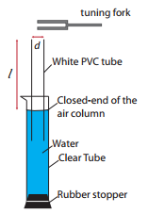Figure 2 – Example of the experiment’s setup (NASA, 2010)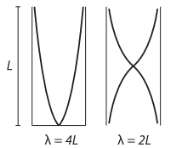Figure 3 – Resonance tube with closed and open end (NASA, 2010)

Although compressions and rarefactions are in opposite directions, when they are reflected at the end of the closed tube (closed end is water) into the converse direction; propagation of waves are produced. The wavelength is calculated through the air column, because a tube’s shortest resonance length is a quarter of the wavelength (Figure 3). Resonance occurs at the greatest amplitude of the sound waves, hence, wavelength can be calculated as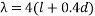(Walding R et al, 2004). The fundamental frequency (first harmonic) has a node (when displacement of the amplitude is zero) at the closed end of the air column and an antinode at the open end (Figure 3 left) (The Physics Classroom, 1996-2015). By adjusting the tube, the third harmonic will appear next as one vibrational node and two vibrational antinodes form the frequency of the harmonic (Figure 3 right). Even though the wavelength and frequency can be multiplied to find the velocity of sound, they are not factors that will affect the velocity, which indicates the changes in wavelength only results in an inverse impact of the frequency (The Physics Classroom, 1996-2015).

4.0 Discussion

4.1 Analysis

By investigating the accuracy of the air-column length using resonance tubes in water, it was clear that the results were achieved with relatively high accuracy when compared with the calculated air-column length when the room temperature was measured and assumed to be constant at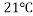; hence the velocity of sound was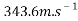. It was discovered that the resonance tube’s diameter had an indirect relationship with the length of air-column, which an increase in tube’s diameter results in a decrease in the air-column length. From Table 1, the air-column lengths for each tube at all frequency declined from 0.161m, 0.231m and 0.329m to 0.134m, 0.203m and 0.301m respectively as the diameter of tubes increased from 0.0164m to 0.0865m (same trend in Table 2). As Graph 1 showed more explicitly through the decreasing linear trendlines at different frequencies, the indirect relationship between air-column length and tube diameter was justified. Moreover, the gradients of the trendlines were the end correction that appeared to be between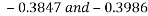, which approximately corresponded with equations of the theoretical data and the formula of wavelength (), but for this experiment using end correction of 0.4 could be invalid as each set of data may have its specific end correction, possibly causing inaccuracies. The measured data seemed to contain relatively high accuracy (overlapped with the theoretical data) in contrast with the theoretical data (Table 2) in Graph 1. Therefore, the end correction and hypothesis were justified.

As frequency is inversely proportional to wavelength, Graph 2 illustrated the inverse relationship between frequency and air-column length where all air-column lengths declined as the frequency grew. While the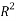values were close to 1, the data plotted did not perfectly fit the trendlines, which indicated that some errors were in the data (see 4.2 Evaluation). Due to tube B and C having similar diameters (0.038m and 0.039m), and only three decimal places were kept when calculating the air-column lengths, the trends were not presented distinctly as the other three tubes. If the results were kept in more decimal places, tube C would have a shorter air-column length than tube B at constant velocity of sound and frequency.

Anomalies were expected and four were identified (highlighted in Table 7). Standard deviation was used to distinguish between the raw data. Results were seen as anomalies if they were not in the range of two standard deviations and average air-column length (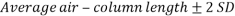). The highlighted data were believed to be one-off values as they appeared randomly, hence disregarded when calculating the average air-column length. The anomalies may have occurred due to parallax when interpreting the measurements from the measuring tape, which can be avoided in further experiments. Another reason may also be mistaking overtones as resonance or missing points of resonance, and particles (e.g. PVC pipe’s debris/scraps) Overall, the data collected were relatively reliable and expected in the hypothesis, thus, justified the inverse relationships between air-column length and tube, as well as frequency and air-column. Therefore, the data proved that wavelength was inversely proportional to frequency (The Physics Classroom, 1996-2015).

4.2 Evaluation

The experiment was completed in one day with consistency. However, errors were found between measured data and theoretical data. Table 3 showed the percentage error between measured and calculated (theoretical) air-column length. All percentage errors were less than 1% (varied from 0.004% to 0.612%). Hence, the errors were insignificant and the measured results contained relatively high accuracy with precision. Theoretically, the velocity of sound should be the same at across all tubes at all frequencies as the temperature was kept constant; however, the data varied between a range of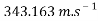and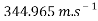(Table 4). Percentage errors were negligible (all under 1% with highest value of 0.397%) which were calculated to compare with the theoretical velocity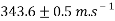at(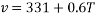) (Table 5). Propagation error of velocity of sound was also investigated by considering the error of apparatus, which was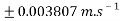compared with the theoretical velocity. Since this error was less than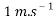, the error was considered to be insignificant that the measured data contained comparatively high accuracy; thus, justified the experiment as a valid experiment. Graph 3 presented the average velocity calculated, the velocities of five pipes differed from each other. Theoretically, an increasing trend in variance should present in Graph 3, but pipe B and C seems to have extremely large variances (errors), hence disregarded. Thus, increasing trend only applies to pipe A, C and E. Evidently, pipe A had the smallest variance, which implied high precision; hence, pipe A was considered to have the highest accuracy.

Furthermore, random errors were made as the tuning fork may have been held incorrectly as hands were moving up and down to adjust the air-column, or pipes were not held completely perpendicular to the ground. The anomalies can be improved by using an electronic ruler to collect air-column lengths to avoid parallax. When finding the harmonics, overtones which relates to the third and fifth frequencies should be avoided. In addition, room temperature may be measured when collecting each trial’s data to calculate the speed of sound to ensure higher accuracy of the air-column length. Due to the experiment being conducted in the classroom with all other experiments conducting at the same time, surrounding noise might be another reason for anomalies occurring; hence the experiment should be completed in a quiet room to avoid resonance interference by other sounds. Furthermore, the experiment can be extended by using AFO (Audio Frequency Oscillator) that generates frequency (at certain level) and a speaker instead of tuning forks to alternate the frequency and water level to determine the air-column length (Gadani D.H, 2011).

Our academic experts are ready and waiting to assist with any writing project you may have. From simple essay plans, through to full dissertations, you can guarantee we have a service perfectly matched to your needs.

Resonance is used in real life applications, usually for music instruments. Flutes can be estimated as cylindrical tubes with two open ends, whereas a clarinet has a closed end which the air is blown from one end and resonance occurs (Walding R et al, 2004). Clarinets usually have frequency range between 125Hz to 2000Hz (Brown S, 1996-2012) with 15mm inner diameter (Fox S, 2000). Since clarinet’s frequency could be around 200Hz, theoretical equation (at 256Hz from Graph 1) was manipulated (Graph 4) to determine the air-column length of the clarinet. The fundamental harmonic is predicted to occur at 33cm; however, at the third harmonic the result (99cm) almost matches with the real clarinet length of 94cm (Nave R, 2015). The length of clarinet must be an exact wavelength in order for the resonance to occur. Therefore, with a percentage error of 6.38% the prediction seems reasonable and appropriate, which justifies that the experiment can be extrapolated to real life application in the future.

5.0 Conclusion

When the velocity of sound was kept constant at a certain temperature, the resonance tubes’ inner diameters were measured with known frequencies written on tuning forks, the wavelength was calculated, hence the air-column length could be determined (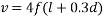). It was found that the air-column length had an indirect relationship with the frequency when velocity of sound was kept constant and an inverse relationship with the tubes’ diameters while both velocity of sound and frequency were maintained the same. This supported the hypothesis that frequencies would have similar impacts on the air-column as the decrease in frequency would lead to an increase in air-column length when diameter was unchanged.

View all

## DMCA / Removal Request

If you are the original writer of this essay and no longer wish to have your work published on UKEssays.com then please:

Related Services

Prices from

KD47

Approximate costs for:

• 1000 words
• 7 day delivery

Related Lectures